# 2D slice from extruded polygon after substraction

• Hello

First of all, would like to thank the developers who made this framework possible.

I am starting off with a linearly extruded polygon and then subtract a cube out of it.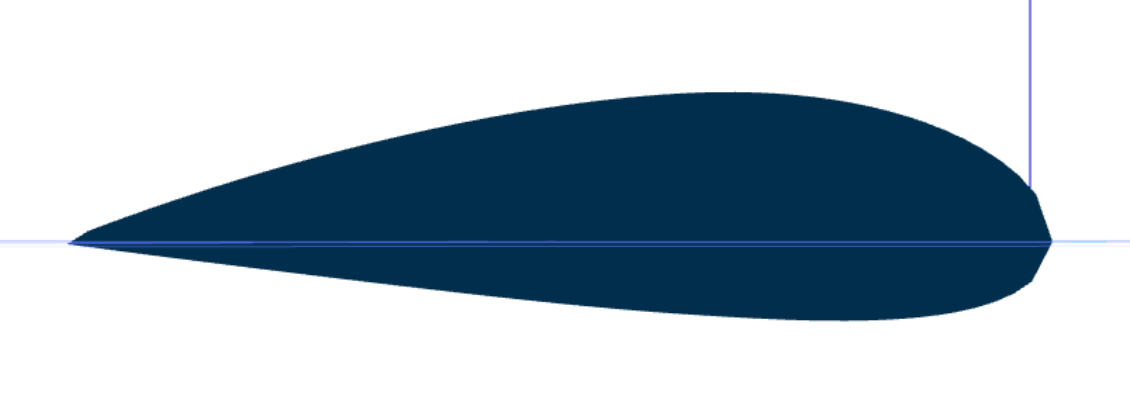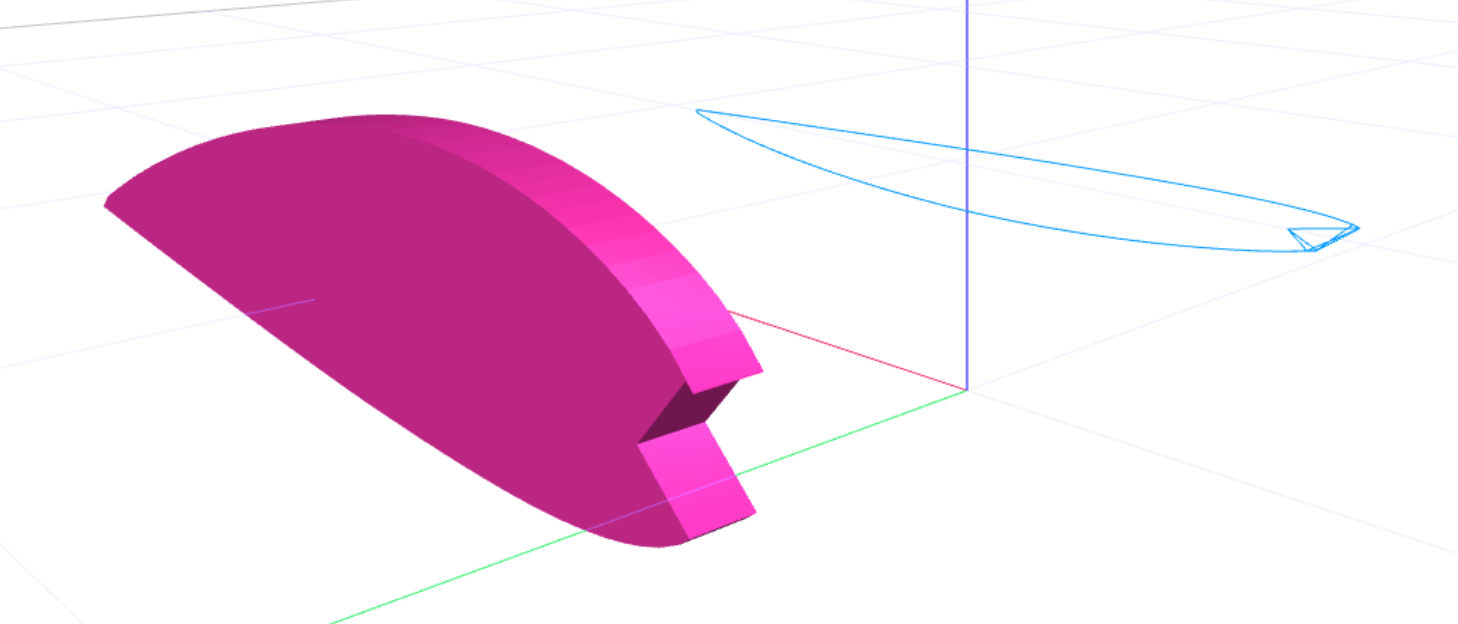Then I want to get a 2d slice in vertical plane. To achieve this I am going through all polygons and all vertices from a very thin slice ignoring all polygons with same z-axis (walls) and extract the x,y and ignore the z altogether.

``````this.profile3D.polygons.forEach(function (polygon, index) {
let local_this2 = local_this

polygon.vertices.forEach(function (v, i) {
let av_arr = polygon.vertices.map(function (x) {
return parseInt(x as any)
}) as Array<number>

let is_vertical = new Set(av_arr).size < 2
if (!is_vertical) {
local_this2.profile2D.push([v, v])
}
})
})
}
``````

Finally I sort of get the 2d slice but with some points "tangled" (shape front end)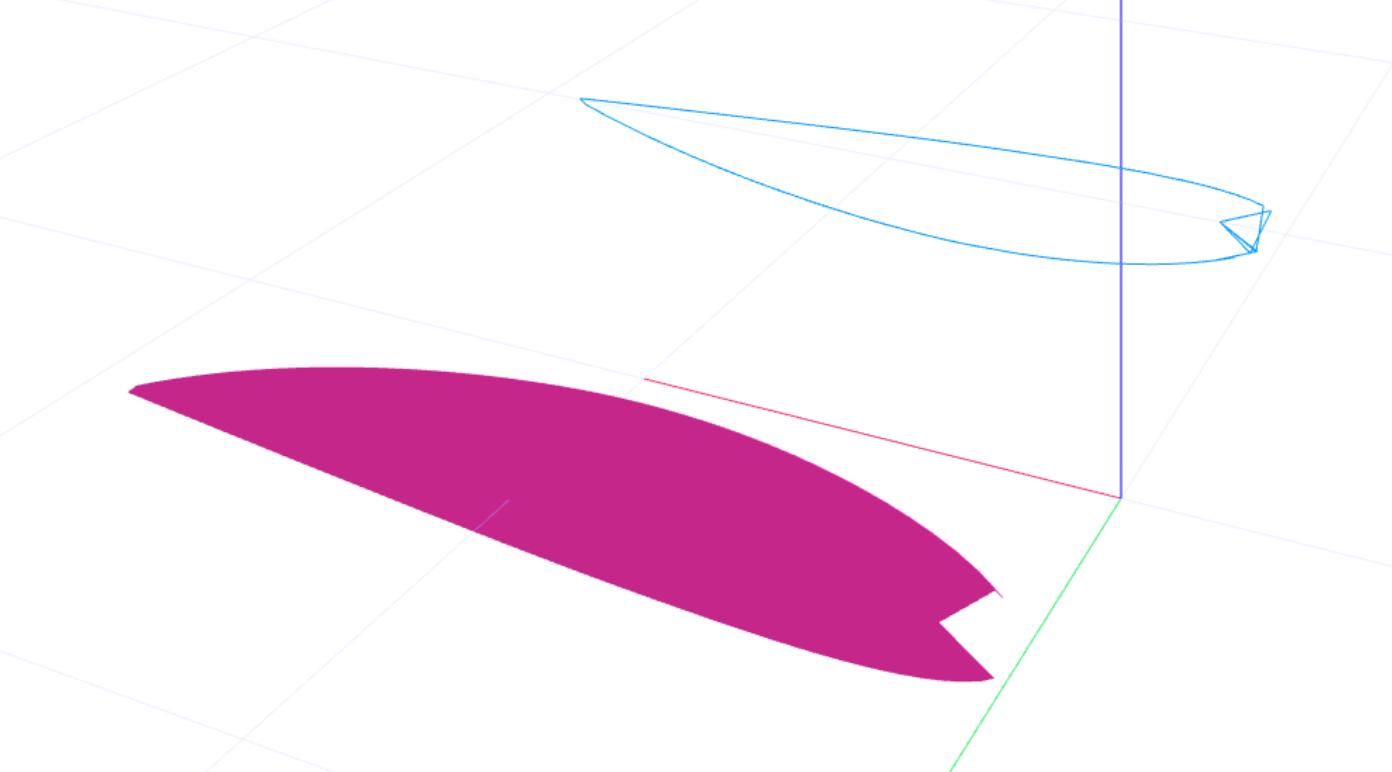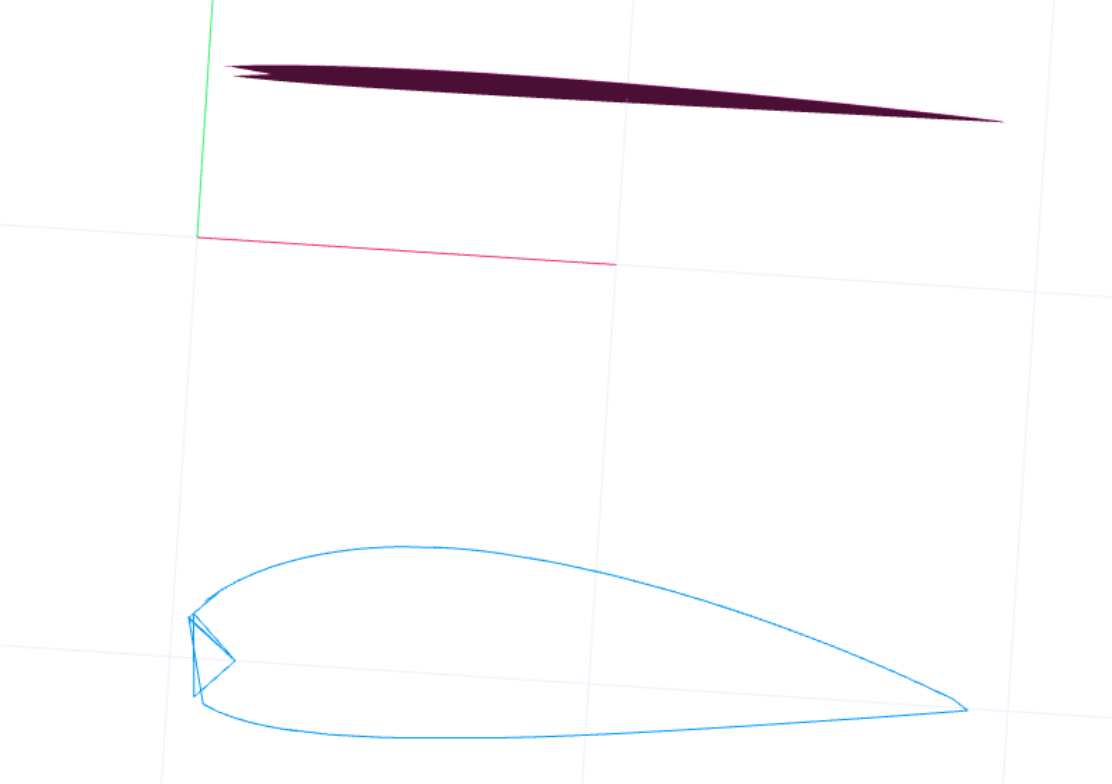How can I make sense of the mesh's structure to be able to walk the mesh so points are in order and correctly joined?
I've excluded the "walls". The mesh seems to be a 4 pct polygon and sometimes a 3 point polygon. More complex shapes turn out more tangled points.
Is there a common layout of the mesh that I can lookup somewhere else? I have tried checkout out the code but could not figure it out.

I have also tried extracting the x,y of any 2 corners of the 4 pct polygon which are not "vertical walls/sides"

``````this.profile3D.polygons.forEach(function (polygon, index) {
let local_this2 = local_this

polygon.vertices.forEach(function (v, i) {
let av_arr = polygon.vertices.map(function (x) {
return parseInt(x as any)
}) as Array<number>

let is_vertical = new Set(av_arr).size < 2
if (!is_vertical) {
local_this2.profile2D.push([polygon.vertices, polygon.vertices])
}
})
})
}
``````

Artefacts like this started to come out but no luck with any combination.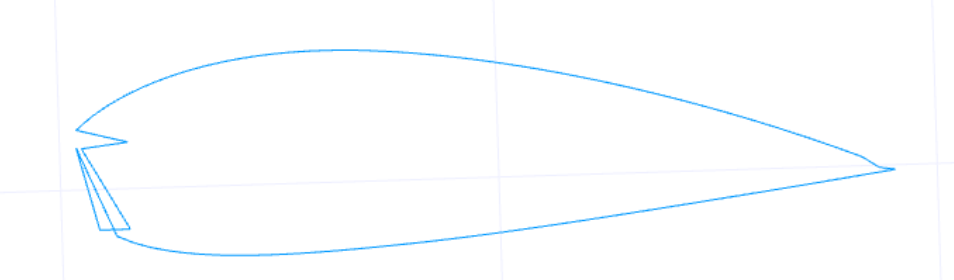Any suggestions are appreciated. Thanks!
P.S Wish my skills would be good enough to contribute.

• @Ion-0 That's great !

• @gilboonet Thanks for the response. I believe you mean the "sides" are rendered using polygons. indeed they are lots of polygons. I might give that a try using the 2d constructive geometry. i've gotten the result right using the fromfakepolygons routine.

• @z3dev
I have got it working using the routine pointed at by you. it was a bit confusing as it throws error for polygons with more than "2 dimension" and the axis was different.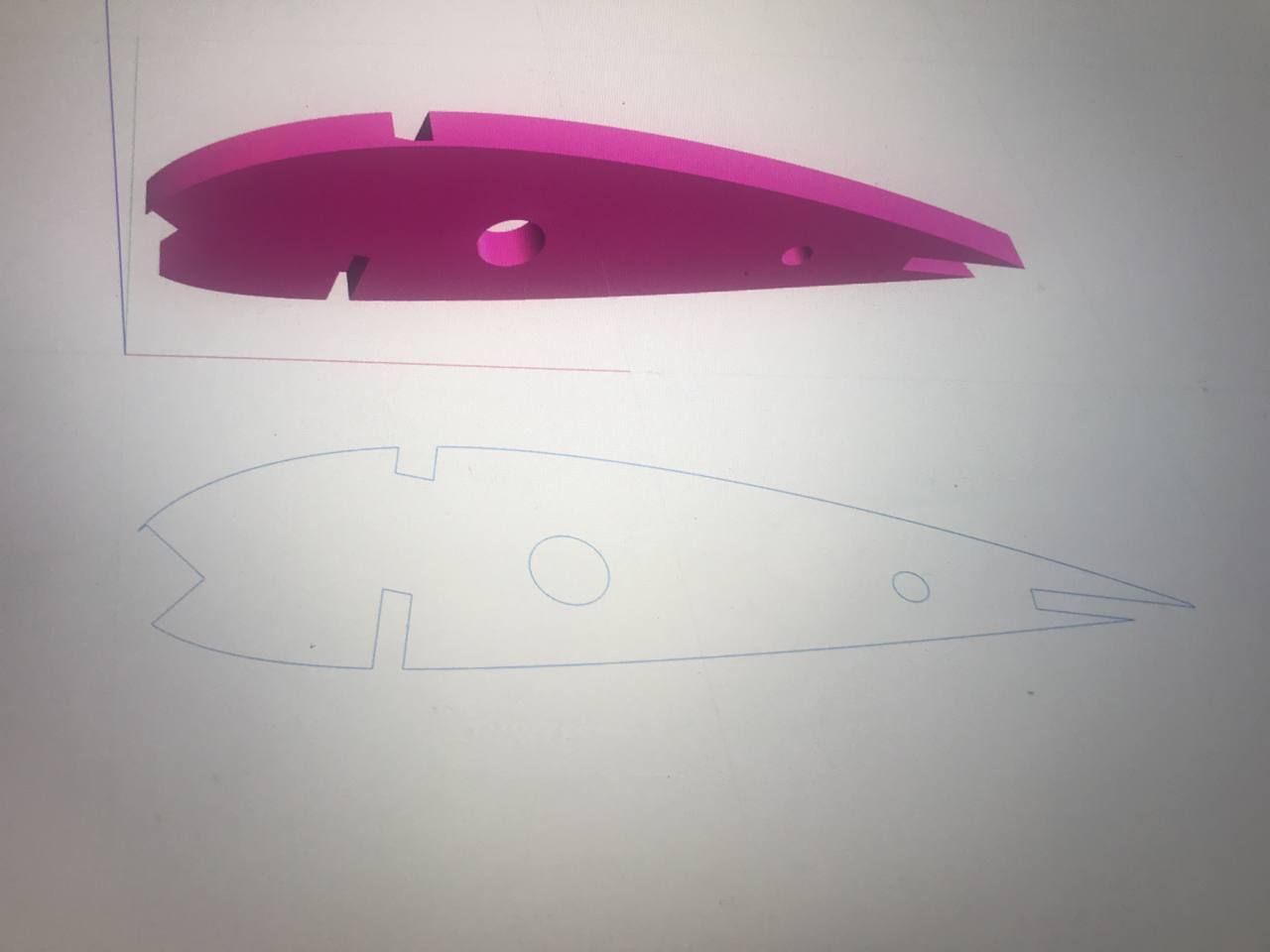• @Ion-0 If I approximate your design, and get a slice of it, I have a set of 3 polygons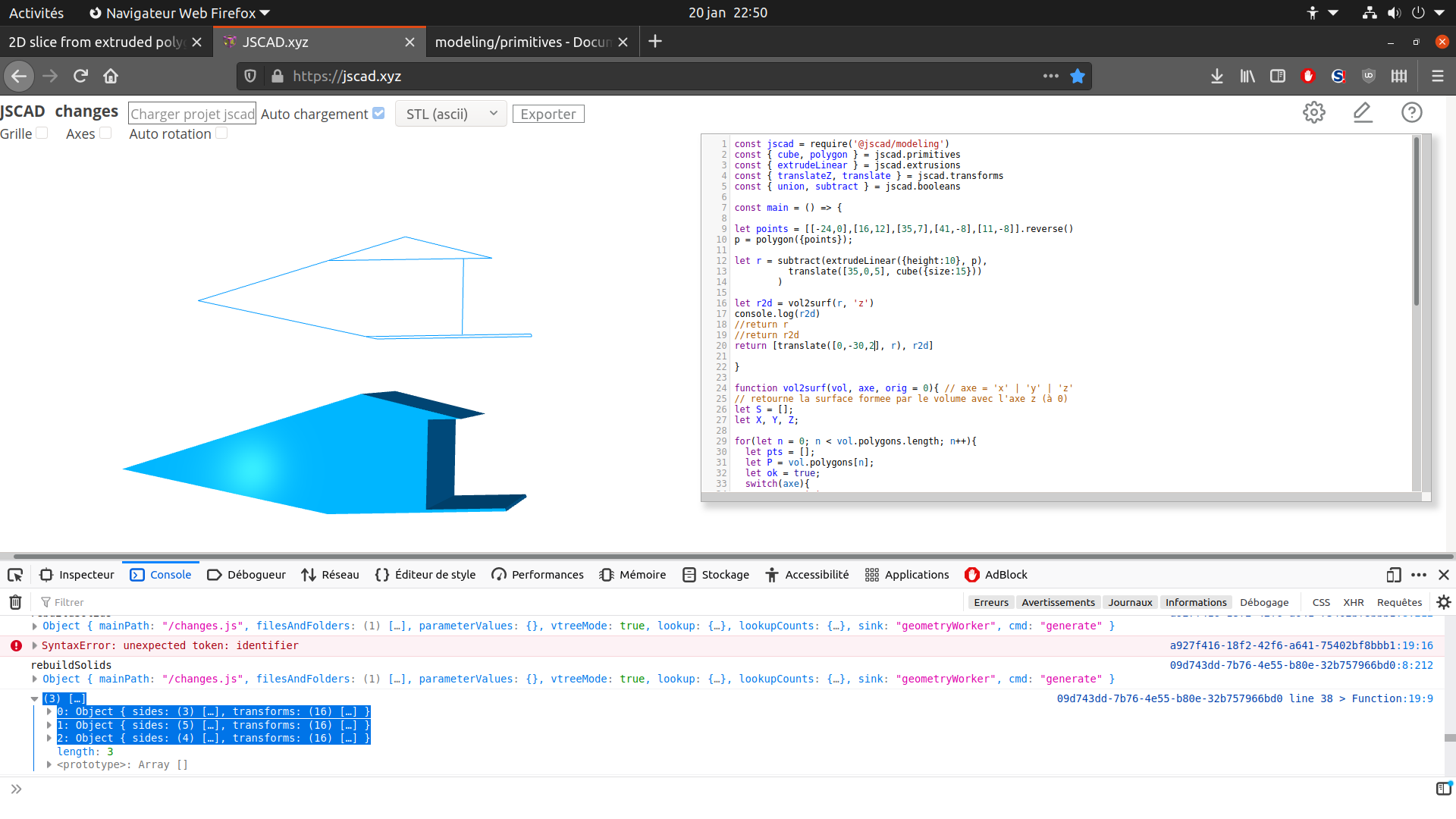• @z3dev thanks!

• @gilboonet The whole 2d contour is one single polygon of points. where should i get multiple polygons from? from the sides?

• Hello, did you try to unify the polygons ? I have some code that does the same kind of thin slicing and the 2d slice is an union of the polygons.

• @Ion Welcome!

You are already doing things that 'normal' designers don't attempt.There's a piece of code that does a very simular thing, converting 3D polgons (from boolean operations) to 2D sides. This might help you to understand a little more about the 2D geometry (geom2).

https://github.com/jscad/OpenJSCAD.org/blob/V2/packages/modeling/src/operations/booleans/fromFakePolygons.js